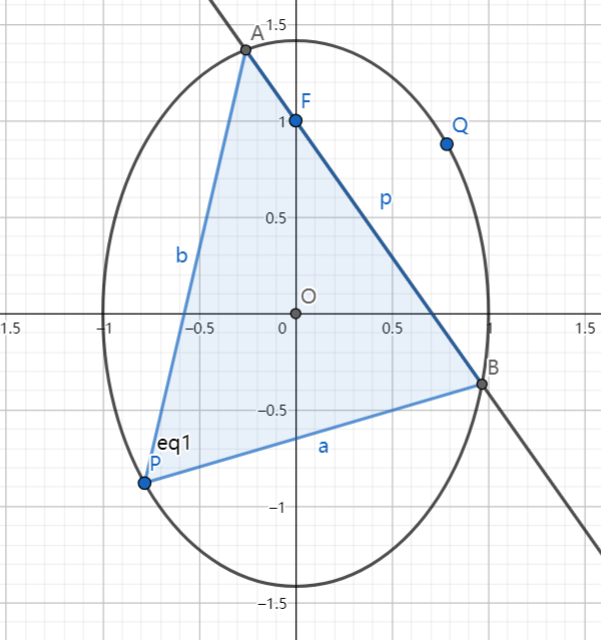# SAT1000 - P838

Geometry Level pendingAs shown above, the ellipse has equation: $x^2+\dfrac{y^2}{2}=1$, $F(0,1)$, line $l: y=-\sqrt{2} x + 1$ intersects with the ellipse at point $A,B$, and $P$ is a point on the ellipse so that $\overrightarrow{OA}+\overrightarrow{OB}+\overrightarrow{OP}=\textbf 0$.

Point $P,Q$ are symmetry about point $O$, and it turns out $A,P,B,Q$ are on the same circle.

The radius of the circle is $r$. Submit $\lfloor 1000r \rfloor$.

Have a look at my problem set: SAT 1000 problems

×